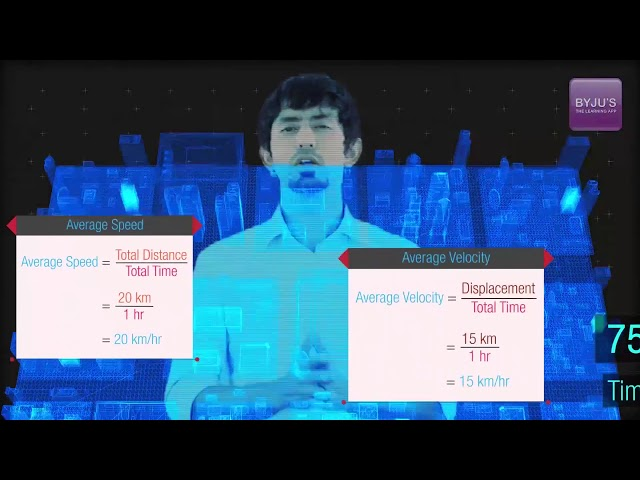# Speed And Its Units

Measurement is the most important concept of science. There are many measurable quantities which are measured in base or physical fundamental units. Speed is one such measurable quantity that measures the ratio of the distance travelled by an object to the time required to travel that distance. In this session, let us learn in detail about speed.

## What is Speed?

Speed is defined as

The rate of change of position of an object in any direction.

Speed is measured as the ratio of distance to the time in which the distance was covered. Speed is a scalar quantity as it has only direction and no magnitude.

## Speed Formula

The formula of speed is given in the table below:

 $$\begin{array}{l}s= \frac{d}{t}\end{array}$$

Where,

• s is the speed in m.s-1
• d is the distance traveled in m
• t is the time taken in s## Speed Unit

Following are the units of speed are:

 CGS system cm.s-1 SI system ms-1

### Finding the Dimensional Formula of Speed

The mathematical representation of speed is:

$$\begin{array}{l}Speed = \frac{Distance}{Time}\end{array}$$

Dimensional formula of Distance = M0L1T0

Dimensional formula of time = M0L0T1

Dividing the dimensional formula of distance by the dimensional formula of time, we get:

$$\begin{array}{l}\frac{M^0L^1T^0}{M^0L^0T^1}=M^0L^0T^{-1}\end{array}$$

Therefore, the dimensional formula of speed =

M0L1T-1

## Types of Speed

There are four types of speed and they are:

• Uniform speed
• Variable speed
• Average speed
• Instantaneous speed

Uniform speed: A object is said to be in uniform speed when the object covers equal distance in equal time intervals.

Variable speed: A object is said to be in variable speed when the object covers a different distance at equal intervals of times.

Average speed: Average speed is defined as the uniform speed which is given by the ratio of total distance travelled by an object to the total time taken by the object.

Instantaneous speed: When an object is moving with variable speed, then the speed of that object at any instant of time is known as instantaneous speed.

## Measurement of Speed

For the measurement of speed in vehicles, speedometers are used. To measure the distance covered odometers are used. Speed can also be calculated with the help of a graph. The Distance-time graph helps in understanding the speed of an object.

## Frequently Asked Questions – FAQs

### What is the SI unit of speed?

The SI unit of speed is ms-1.

### What is the formula to find the speed?

Speed = Distance/Time

### What are the types of speed?

Types of speed are:

• Uniform speed
• Variable speed
• Average speed
• Instantaneous speed
• ### Define speed.

The ratio of distance to the time in which the distance was covered defines the speed.

### What is uniform speed?

When an object covers equal distance in equal time intervals it is said to be in uniform speed.

Stay tuned with BYJU’S to learn more interesting science topics with engaging videos!

Test your Knowledge on Measurement Of Speed

#### 1 Comment

1. Priya Chauhan

Very nice very good